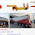We were discussing “Area moment of inertia” and “The difference between centroid and centre of gravity”,” and also we have seen the “Basic principle of complementary shear stresses” with the help of our previous posts.

Now we are going further to start our discussion to understand the basic concept of radius of gyration with the help of this post.

### Let us first see here the basic concept of radius of gyration

Radius of gyration of a body or a given lamina is basically defined as the distance from the given axis up to a point at which the entire area of the lamina will be considered to be concentrated.

We can also explain the radius of gyration about an axis as a distance that if square of distance will be multiplied with the area of lamina then we will have area moment of inertia of lamina about that given axis.

Let us see the following figure which indicates one lamina with area A. Let us assume that lamina, displayed here, is made with number of small elemental areas a1, a2, a3, a4 …etc. As we have considered above that the total area of the lamina is A, now we need to determine here the radius of gyration about an axis for the given lamina.
Where,
x1= Distance of the C.G of the area a1 from OY axis
x2= Distance of the C.G of the area a2 from OY axis
x3= Distance of the C.G of the area a3 from OY axis
x4= Distance of the C.G of the area a4 from OY axis

Similarly, we will have
y1= Distance of the C.G of the area a1 from OX axis
y2= Distance of the C.G of the area a2 from OX axis
y3= Distance of the C.G of the area a3 from OX axis
y4= Distance of the C.G of the area a4 from OX axis
Area moment of inertia about the OY axis = a1.x12 + a2.x22 + a3.x32+ a4.x42 +…….
Iyy = a1.x12 + a2.x22 + a3.x32+ a4.x42 +…….
Iyy = Σ a.x2

Let us assume that entire area of the given lamina is concentrated at a distance k from the reference axis i.e. OY axis and therefore area moment of inertia for the entire area about the reference axis i.e. OY axis will be A.k2.
Iyy = A.k2

Similarly, Area moment of inertia about the OX axis
Ixx = a1.y12 + a2.y22 + a3.y32+ a4.y42 +…….
Ixx = Σ a.y2

Let us assume that entire area of the given lamina is concentrated at a distance k from the reference axis i.e. OX axis and therefore area moment of inertia for the entire area about the reference axis i.e. OX axis will be A.k2.
Ixx = A.k2

Do you have any suggestions or any amendment required in this post? Please write in comment box.

### Reference:

Strength of material, By R. K. Bansal
1.